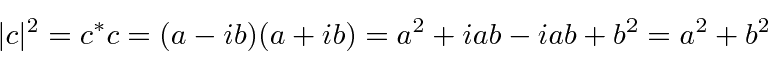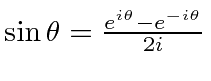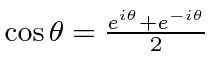### Review of Complex Numbers

This is a simple review, but, you must make sure you use complex numbers correctly. One of the most common mistakes in test problems is to forget to take the complex conjugate when computing a probability.

A complex numberconsists of a real partand an imaginary part. (We chooseandto be real numbers.)is the square root of -1.

The complex conjugate ofis. (Just change the sign of all the.)

The absolute square of a complex number is calculated by multiplying it by its complex conjugate.This give the magnitude squared of the complex number. The absolute square is always real.

We will use complex exponentials all the time.You can verify that the absolute square of these exponentials is always 1. They are often called a phase factor.

We can writeand.

As with other exponentials, we can multiply them by adding the exponents.Jim Branson 2013-04-22